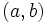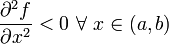# Concave function

## Definition

### For a function of a single variable

Suppose$f$ is a function from an interval$(a,b)$ in the real numbers, to the real numbers. We say that$f$ is a concave function on$(a,b)$ if:$\frac{\partial^2 f}{\partial x^2} \le 0 \ \forall \ x \in (a,b)$.

We say that$f$ is a strictly concave function on$(a,b)$ if:$\frac{\partial^2 f}{\partial x^2} < 0 \ \forall \ x \in (a,b)$.

Concave functions could be of many type:

• Concave functions that are increasing throughout, and in the limit still have positive first derivative.
• Concave functions that are increasing throughout, and in the right limit have zero derivative.
• Concave functions that start off increasing and end up decreasing.
• Concave functions that start off with zero derivative and end up decreasing.
• Concave functions that start off with negative first derivative.

## Facts

### Negative of convex

A function$f$ is concave if and only if the function$-f$ is a convex function. Similarly,$f$ is a strictly concave function if and only if$-f$ is a strictly convex function.

If$f$ is a concave function, and it is invertible, then the inverse function$f^{-1}$ is also a concave function.